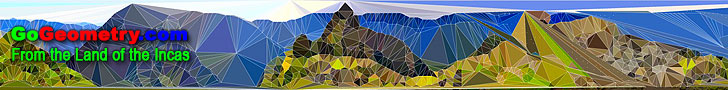# Recent additions (Page 16), Online LearningGo Geometry from the Land of the Incas - Recent Additions (Page 16) Geometry Problem 627 Cyclic Quadrilateral, Congruence, Concyclic Points. Geometry Problem 626 Triangle, Distance from the Incenter to an Excenter. Geometry Problem 625 Triangle, Parallel Lines, Congruence, Angle Bisector. Geometry Problem 624 Triangle, Incenter, Excenter, Incircle, Excircle, Similarity. Geometry Problem 623 Triangle, Incenter, Excenter, Incircle, Excircle, Concyclic Points. Geometry Problem 622 Square with Perpendiculars, Transversal, Congruence. Geometry Problem 621 Three Rectangles, Diagonals, Centers, Angles. Geometry Problem 620 Three Rectangles, Diagonals, Centers, Angles. Geometry Problem 619 Triangle Area, 30 Degrees, Incircle, Incenter, Tangent. Geometry Problem 618 Triangle Area, 60 Degrees, Incircle, Incenter, Tangent. Geometry Problem 617 Right Triangle Area, Incircle, Incenter, Tangent, 90 Degrees. Geometry Problem 616 Parallelogram, Diagonals, Intersecting Lines, Parallel Lines. Geometry Problem 615 Right Triangle with a Square, Diagonals, Center, Area of Quadrilateral. Geometry Problem 614 Parallelogram, Diagonals, Intersecting Lines, Parallel Lines. Geometry Problem 613 Altitude of a Triangle, Perpendicular, Collinear Points. iPad, iPhone Apps: iCrosss, 3D Polyhedron - Video. Application supports such kinds of polyhedra as regular polyhedra  (Platonic solids), Pyramids, Prisms, Anti-prisms and Archimedean solids and duals. Geometry Problem 612 Altitude of a Triangle, Perpendicular, Congruence. Geometry Problem 611 Altitude of a Triangle, Perpendicular, Collinear Points, Parallel. Geometry Problem 610 Intersecting Circles, Radius, Parallel, Common Tangent, Angle, 90 Degrees. Geometry Problem 609 Triangle, Perpendiculars, 90 Degrees, Collinearity. Geometry Problem 608 Square with Right Triangles, Metric Relations, Congruence, Measurement. Geometry Problem 607 Intersecting Circles, Triangles, Metric Relations, Measurement. Geometry Problem 606 Isosceles Triangles, Perpendicular and Parallel Lines. Geometry Problem 605 Circle, Square, Chord, Perpendicular, Circular Segment, Metric Relations. Geometry Problem 604 Parallelograms, Triangle, Trapezoid, Area. Geometry Problem 603 Parallelogram, Diagonals, Congruence, Concurrency. Geometry Problem 602 Triangle, Angles, 30 and 45 Degrees, Cevian, Congruence. Geometry Problem 601 Cyclic Quadrilateral, Incenter, Excenter, Rectangle. Geometry Problem 600 Circle, Area, Tangent, Semicircle, Tangency Point. Geometry Problem 599 Regular Hexagon, Square, Midpoint, Angle, Degrees. Go to Page: Previous | 1 | 2 | 3 | 4 | 5 | 6 | 7 | 8 | 9 | 10 | 11 | 12 | 13 | 14 | 15 | 16 | 17 | 18 | 19 | 20 | 21 | 22 | 23 | 24 | 25 | 26 | 27 | 28 | 29 | 30 | 31 | 32 | 33 | 34 | 35 | 36 | 37 | 38 | 39 | 40 | 41 | 42 | 43 | 44 | 45 | 46 | 47 | 48 | 49 | 50 | Next

 Home | GoGeometry Education | Geometry | Search | Email